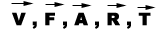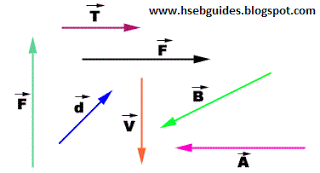## TyroCity

Physics XI Notes for Physics Notes

Posted on • Updated on

# Introduction To Scalar And Vector

Scalar and vector

Scalar quantities
Physical quantities which can completely be specified by a number (magnitude) having an appropriate unit are known as scalar quantities.
Scalar quantities do not need direction for their description.
Scalar quantities are comparable only when they have the same physical dimensions.
Two or more than two scalar quantities measured in the same system of units are equal if they have the same magnitude and sign.
Scalar quantities are denoted by letters in ordinary type.
Scalar quantities are added, subtracted, multiplied or divided by the simple rules of algebra.

Examples:
Work, energy, electric flux, volume, refractive index, time, speed, electric potential, potential difference, viscosity, density, power, mass, distance, temperature, electric charge, electric flux etc.

Vectors quantities

Physical quantities having both magnitude and direction
with appropriate unit are known as vector quantities.

We can’t specify a vector quantity without mention of direction.
vector quantities are expressed by using bold letters with arrow sign such as:vector quantities can not be added, subtracted, multiplied or divided by the simple rules of algebra.
vector quantities added, subtracted, multiplied or divided by the rules of trigonometry and geometry.

Examples:

Velocity, electric field intensity, acceleration, force, momentum, torque, displacement, electric current, weight, angular momentum etc.

Representation of vectors

On paper vector quantities are represented by a straight line with arrow head pointing the direction of vector or terminal point of vector.

A vector quantity is first transformed into a suitable scale and then a line is drawn with the help of the scale chosen in the given direction.